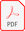## Times Tables from 2 - 15

Times Table Calculator
Given below is the times chart for number 2 - 30. Also you can get a free printable pdf file for the times chart.

Memorizing times chart are very critical for solving mathematical problems and even very helpful in our daily life. We encourage you to memorize times table at least till 30. Read the chart aloud and keep practicing.
Free downloable and printable multiplication table pdf:

Times Chart from 2 - 15Get the multiplication tables from 16 - 30 here: Times Chart from 16 - 30
Times Table of 2

2  ×  1  =  2

2  ×  2  =  4

2  ×  3  =  6

2  ×  4  =  8

2  ×  5  =  10

2  ×  6  =  12

2  ×  7  =  14

2  ×  8  =  16

2  ×  9  =  18

2  ×  10  =  20

2  ×  11  =  22

2  ×  12  =  24

Times Table of 3

3  ×  1  =  3

3  ×  2  =  6

3  ×  3  =  9

3  ×  4  =  12

3  ×  5  =  15

3  ×  6  =  18

3  ×  7  =  21

3  ×  8  =  24

3  ×  9  =  27

3  ×  10  =  30

3  ×  11  =  33

3  ×  12  =  36

Times Table of 4

4  ×  1  =  4

4  ×  2  =  8

4  ×  3  =  12

4  ×  4  =  16

4  ×  5  =  20

4  ×  6  =  24

4  ×  7  =  28

4  ×  8  =  32

4  ×  9  =  36

4  ×  10  =  40

4  ×  11  =  44

4  ×  12  =  48

Times Table of 5

5  ×  1  =  5

5  ×  2  =  10

5  ×  3  =  15

5  ×  4  =  20

5  ×  5  =  25

5  ×  6  =  30

5  ×  7  =  35

5  ×  8  =  40

5  ×  9  =  45

5  ×  10  =  50

5  ×  11  =  55

5  ×  12  =  60

Times Table of 6

6  ×  1  =  6

6  ×  2  =  12

6  ×  3  =  18

6  ×  4  =  24

6  ×  5  =  30

6  ×  6  =  36

6  ×  7  =  42

6  ×  8  =  48

6  ×  9  =  54

6  ×  10  =  60

6  ×  11  =  66

6  ×  12  =  72

Times Table of 7

7  ×  1  =  7

7  ×  2  =  14

7  ×  3  =  21

7  ×  4  =  28

7  ×  5  =  35

7  ×  6  =  42

7  ×  7  =  49

7  ×  8  =  56

7  ×  9  =  63

7  ×  10  =  70

7  ×  11  =  77

7  ×  12  =  84

Times Table of 8

8  ×  1  =  8

8  ×  2  =  16

8  ×  3  =  24

8  ×  4  =  32

8  ×  5  =  40

8  ×  6  =  48

8  ×  7  =  56

8  ×  8  =  64

8  ×  9  =  72

8  ×  10  =  80

8  ×  11  =  88

8  ×  12  =  96

Times Table of 9

9  ×  1  =  9

9  ×  2  =  18

9  ×  3  =  27

9  ×  4  =  36

9  ×  5  =  45

9  ×  6  =  54

9  ×  7  =  63

9  ×  8  =  72

9  ×  9  =  81

9  ×  10  =  90

9  ×  11  =  99

9  ×  12  =  108

Times Table of 10

10  ×  1  =  10

10  ×  2  =  20

10  ×  3  =  30

10  ×  4  =  40

10  ×  5  =  50

10  ×  6  =  60

10  ×  7  =  70

10  ×  8  =  80

10  ×  9  =  90

10  ×  10  =  100

10  ×  11  =  110

10  ×  12  =  120

Times Table of 11

11  ×  1  =  11

11  ×  2  =  22

11  ×  3  =  33

11  ×  4  =  44

11  ×  5  =  55

11  ×  6  =  66

11  ×  7  =  77

11  ×  8  =  88

11  ×  9  =  99

11  ×  10  =  110

11  ×  11  =  121

11  ×  12  =  132

Times Table of 12

12  ×  1  =  12

12  ×  2  =  24

12  ×  3  =  36

12  ×  4  =  48

12  ×  5  =  60

12  ×  6  =  72

12  ×  7  =  84

12  ×  8  =  96

12  ×  9  =  108

12  ×  10  =  120

12  ×  11  =  132

12  ×  12  =  144

Times Table of 13

13  ×  1  =  13

13  ×  2  =  26

13  ×  3  =  39

13  ×  4  =  52

13  ×  5  =  65

13  ×  6  =  78

13  ×  7  =  91

13  ×  8  =  104

13  ×  9  =  117

13  ×  10  =  130

13  ×  11  =  143

13  ×  12  =  156

Times Table of 14

14  ×  1  =  14

14  ×  2  =  28

14  ×  3  =  42

14  ×  4  =  56

14  ×  5  =  70

14  ×  6  =  84

14  ×  7  =  98

14  ×  8  =  112

14  ×  9  =  126

14  ×  10  =  140

14  ×  11  =  154

14  ×  12  =  168

Times Table of 15

15  ×  1  =  15

15  ×  2  =  30

15  ×  3  =  45

15  ×  4  =  60

15  ×  5  =  75

15  ×  6  =  90

15  ×  7  =  105

15  ×  8  =  120

15  ×  9  =  135

15  ×  10  =  150

15  ×  11  =  165

15  ×  12  =  180